Thank you for visiting nature.com. You are using a browser version with limited support for CSS. To obtain the best experience, we recommend you use a more up to date browser (or turn off compatibility mode in Internet Explorer). In the meantime, to ensure continued support, we are displaying the site without styles and JavaScript.

# A Biologically Inspired Network Design Model

## Abstract

A network design problem is to select a subset of links in a transport network that satisfy passengers or cargo transportation demands while minimizing the overall costs of the transportation. We propose a mathematical model of the foraging behaviour of slime mould P. polycephalum to solve the network design problem and construct optimal transport networks. In our algorithm, a traffic flow between any two cities is estimated using a gravity model. The flow is imitated by the model of the slime mould. The algorithm model converges to a steady state, which represents a solution of the problem. We validate our approach on examples of major transport networks in Mexico and China. By comparing networks developed in our approach with the man-made highways, networks developed by the slime mould and a cellular automata model inspired by slime mould, we demonstrate the flexibility and efficiency of our approach.

## Introduction

Transport networks are vital infrastructure of human society. Many networks are overloaded and often choked with traffic. Governments in most countries aim to ease congestion by imposing pollution charges1,2, tradable travel credits for congestion management3, parking permits distribution and trading4. Such policies reduce the congestion to some extent, but they also lead to additional problems. For example, in the policy of tradable travel credits for congestion management, it might be complicated to make a decision on how to allocate the limited tradable travel credits to users, how many tradable travel credits should be provided, etc5,6,7. One of the most promising solutions to this problem would be to design an efficient transit network for a transportation system. The efficient transit network is capable of maximizing throughout capacity and minimizing the overall costs8,9,10,11,12,13,14.

The network design problem (NDP) is one of the most challenging transport problems. The problem is defined as follows. Given a weighted graph G, we want to select such a subgraph in G that it satisfies the given point-to-point demand on a transportation and minimizes the overall costs of the transportation. In the past decades, various approaches have been presented to address this issue. The solutions can be divided into two categories: exact solutions15,16,17 and heuristic solutions18,19,20,21. Exact solution methods can deal with NDP in a rigorous manner. However, they are inefficient when dealing with large-scale real-world networks18,22,23. Heuristic approaches, emerged in the past decades24,25, provide approximate yet efficient solutions. The heuristic approaches can tackle a real-world problems with a large number of design variables26,27 and therefore these approaches are more popular than exact solutions20,28,29,30,31.

When we design a transportation network we should, ideally, make it fault tolerant, capable to cope with traffic accidents, terrorist attack and emergency road maintenance. Fault tolerance and high performance attract higher building and maintenance costs. How to make a tradeoff between the overall cost, the fault tolerance and the performance is the problem worthwhile to investigate32,33,34. During their evolution living creatures optimised their transportation networks over million of years: vascular systems of plants and animals35,36, foraging patterns of social insects37,38 migration trails by birds and animals, hunting routes of predators. It is therefore often very fruitful to apply natural solutions in designs of human-made artifacts39.

There is a unique creature which exhibits properties of internal and external living transport systems. This is a acellular slime mould Physarum polycephalum. Plasmodium is a vegetative stage of acellular slime mould P. polycephalum, a syncytium, a single cell with many nuclei, which feeds on microscopic particles40. When foraging for its food the plasmodium propagates towards sources of food particles, surrounds them, secretes enzymes and digests the food. Typically, the plasmodium forms a congregation of protoplasm covering the food source. When several sources of nutrients are scattered in the plasmodium’s range, the plasmodium forms a network of protoplasmic tubes connecting the masses of protoplasm at the food sources.

During its foraging behaviour the plasmodium spans scattered sources of nutrients with a network of protoplasmic tubes. The protoplasmic network is optimized to cover all sources of food and to provide a robust and speedy transportation of nutrients and metabolites in the plasmodium body. The plasmodium’s foraging behaviour can be interpreted as a computation, where data are represented by spatial configurations of attractants and repellents and results of the computation are protoplasmic network formed by the plasmodium on the data sets41,42,43. The problems solved by plasmodium of P. polycephalum include the shortest path41,42, connecting different arrays of food sources in an efficient manner44, implementation of storage modification machines45, Voronoi diagram46, Delaunay triangulation43, logical computing47 and process algebra48, see overview in43.

A mathematical model of Physarum morphological behavior was proposed in49. Bonifaci et al.50 demonstrated that Physarum converges to a shortest path in the network regardless of the initial structure of the network or of the initial mass distribution. In the present paper, we explore Physarum to solve an NDP in terms of costs, efficiency and robustness. We employ the gravity model51 to estimate the traffic flow between a pair of cities. Based on a specific travel demand, we employ Physarum to simulate the transport flow between the cities. Then we allow the slime mould to colonise all cities, develop its protoplasmic network and settle down in some stable sate. The stable state represents a solution of the NDP.

## Results

We will evaluate the networks using measures of cost, efficiency and robustness. In Physaurm algorithm, a threshold value δ as shown in the supplementary material is required to stop the execution of this program. If the threshold value δ is too small, it will take the Physarum algorithm a lot of time to converge to a solution. If δ is too large, the results will not reflect the features of the formulated networks. Here, we adopt a comprising strategy between the execution time of the algorithm and the characteristics of the formulated networks. The threshold value δ is set to be 0.01.

A cost (TL) is the sum of the length of all the edges existing in each network while the length is a representative of geographical distance. We have normalized the cost TL to the total length of the Minimum Spanning Tree (MST) for the corresponding networks. Efficiency (MD) is the transportation performance of each network, which is measured as the sum of minimum distance (MD) between all pairs of nodes. The efficiency MD is also normalized to the sum MDMST of minimum distances between all pairs of nodes in the Minimum Spanning Tree. Finally, the fault tolerance, or robustness, of a network is measured as the probability of the network to become disconnected when a single link is removed. Here, the disconnection is defined as follows: for any pair of nodes, if there is no feasible path between them, we can say the network is disconnected. For example, in MST, the removal of any link will lead to the disconnection of the network.

### Application to Mexican Highways Network

To compare our algorithm with the real slime mould, we have used the results from our previous experimental laboratory studies52. We have selected 19 most populated urban areas shown in Fig. 1(a). The general data on these cities are described in Table 1. Figure 1(b) shows the minimum spanning tree (MST) of Mexico highways.

According to Eq. (8) and the data shown in Table 1, we can construct the basic traffic flow among any two cities. For the row with more than one economic power shown in Table 1, we can regard it as a single row by summing these economic powers, then the corresponding traffic flow can be determined. Specifically speaking, for Row 14, the economic power will be 2 + 1 + 7 = 10. According to Eq. (8), we can figure out the traffic flows from this city to the other cities.

The final step is to construct the network according to the conductivity value α associated with each edge. α is just used to filter out the edges with conductivity less than α. α's values are determined as follows. When the Physarum algorithm is over, we will make full use of this parameter to build the networks with different topologies. Every time, these three parameters (Cost, Performance and Efficiency) change, α will be recorded. We keep recording α's values until the network becomes disconnected. Generally speaking, α's values can reflect the changing trend of these formulated networks. With the increase of α, the less important edges will gradually fade out, which will affect the performance of the formulated network further. In this paper, we set the starting value for α is 0.01. For example, Fig. 2 shows four networks generated by Physarum when α is 0.01, 0.05, 0.16, 0.26, respectively.

Let us now compare the transport performance, fault tolerance and efficiency of each network. The real Mexico highways and the network developed by Physarum are shown in Fig. 3. ue to the reason of the copyright, we cannot show the structure of the real Mexico highways here. But it can be obtained from Ref. 52, which is Fig. 7(a) in Ref. 52. We also compare our results with a cellular automata model, inspired by slime mould, proposed by Tsompanas et al.53. The cellular automata model employs an attractant diffusion equation to describe the foraging behavior of the plasmodium and to calculate the propagation of chemoattractants produced by the nutrient sources. The diffusion of chemoattractants is uniform, while the growth of the slime mould is affected by the concentration of chemoattractants53,54. Note here that the parameters of the model are the same as used in54. Moreover, the input data of the model is only the configuration of the cities in the country and its borders. No economical or population factors are taken into consideration.

Figure 4(a) shows the Mexico highway networks built by Tsompanas’s model. The numbers near blue circles denote the α's value associated with each formulated network. In terms of the transportation performance, the cost of Mexican highways built by the cellular automata model is least among all the alternative networks, which is greater than the MST by a factor of 1.2. Regarding the network performance, the lower its value is, the better the performance is. However, for the network build by the cellular automata model, its transportation performance is about 0.97, which is the worst in all the networks. For the network built by real Physarum, it can be seen that it has a factor of 3.8 when compared with the MST. As for the network formulated by the Physarum algorithm, when α’s value ranges from 0.01 to 0.26, the transportation performance fluctuates gradually. Among them, the networks have less cost but high transportation performance when α ranges from 0.05 to 0.26. This demonstrates that the proposed methods are flexible. In a real-world environment, we can determine the topology of the network and the value αaccording to the specific budget. It can be noted that when α changes from 0.05 to 0.26, the Physarum algorithm can build networks with higher performance but lower cost when compared with other networks. In summary, the Physarum algorithm can achieve better and flexible results with marginally lower costs.

Our Physarum algorithm also outperforms other methods in terms of fault tolerance (FT). As can be seen in Fig. 5(B), the network generated by the cellular automate model has the lowest fault tolerance about 0.3, which means about 70% of faults in this network will lead to the disconnection of any part. However, both the network constructed by real Physarum and the Physarum algorithm have high fault tolerance. When α is 0.01, 0.05, 0.1, 0.16, the formulated networks show the best fault tolerance, which is equal to the maximum tolerance to a random failure of a single link. With the increase of α, fault tolerance of a network decreases gradually. As for the network grown by real Physarum, its fault tolerance is still higher than Mexican highways, which is about 0.9787.

The trade-off between the cost and fault tolerance is measured by FT/TLMST. Mexican highways have a high efficiency, which is about 0.42. For the real Physarum, it has a factor of 0.25. As for the networks formulated by the Physarum algorithm, the efficiency factor becomes the highest when α is 0.26. When α has different values (in other words, when the cost is different), the Physarum takes different measures to adapt to these environments spontaneously. For the cellular automate-based network, the corresponding value is 0.25, which is the third lowest value for the efficiency indicator among all the alternative models.

### Application to China Motorways Network

In China motorways network, we choose 31 most populated major urban areas approximately corresponding to distribution of population densities by 201055 and they are shown in Fig. 6(a). Figure. 6(b) shows the minimum spanning tree of China motorways network. Table 2 displays the population and economic power of each city.

Similarly, we can develop the networks by filtering out the edges with conductivity less than α. Figure 7 shows us the networks for α is 0.02, 0.05, 0.07 and 0.09, respectively. As can be seen in Fig. 7, with the increase of α, some unimportant edges gradually disappear while the critical links are retained. The thickness of every edge reflects the actual size of the traffic flow between different cities. It can be seen that most of the edges with bigger traffic flow are mainly distributed in the central and southeastern China.

Figure 8 shows the slime mould approximation of transport network in China and the real motorways graph, respectively. Let us compare the transport performance, fault tolerance and the efficiency of each network. The motorway network shown in Fig. 9(A) has highest cost and lowest performance. China motorways cost is bigger than MST by a factor of 7. The minimum distance between all pairs of nodes in the motorway network is higher than MST by about 25% percent. Both the networks developed by real Physarum and the proposed Physarum algorithm have less cost. When α is 0.01, Physarum algorithm has more cost when compared with the network built by real Physarum but its performance is better than the performance of the real Physarum. In addition, with the increase of α, the performance of every network constructed by Physarum algorithm decreases gradually while its cost reduces by a larger size. For the cellular automata based network, the constructed network has lowest cost but the performance is the second worst in all the alternatives.

As for the fault tolerance of each network, from Fig. 9, it can be noted that although the cost of China motorways is very high, its fault tolerance is still lower when compared with the network formulated by real Physarum and the network built by Physarum algorithm when α = 0.01. With the change of α, the fault tolerance of the networks built by Physarum algorithm changes a little when compared with the change of its cost. While the network formulated by the cellular automate model has the lowest cost, its fault tolerance is also lowest among all alternatives. The fault tolerance requires a presence of redundant links in the network therefore it increases the cost of the network.

Finally, let us focus on the efficiency of every network. For α = 0.1 the network has the highest efficiency. When α changes, the cost’s change amplitude is greater than the fault tolerance’s. Among these networks, China motorways has the lowest efficiency while the network formulated by real Physarum is the third lowest. Although the network formulated by the cellular automata model has the highest efficiency, both the performance and the fault tolerance of this network is very worse. In the real-world transportation systems, we need to account for all the three parameters. From this point of view, all the above may suggest that the networks formulated by Physarum algorithm are highly efficient. Thus, it is useful to implement this method into real-world applications, such as the transportation network design.

## Discussion

We applied a model of foraging behaviour of slime mould Physarum polycephalum to solve a network design problem by maximising transport capacity of the network and minimising the size and length of the network. The Physarum algorithm solved the network design problem by developing competition between transport routes: the links with high transportation loads increase their conductivity while less used links are removed. We demonstrated the efficiency of the proposed algorithm by comparing networks produced by the Physarum algorithm with networks of man-made highway network in Mexico and motorways networks in China and protoplasmic transport networks grown by the slime mould on a map of major urban areas of Mexico and China and a Physarum-inspired cellular automate model. The networks were compared in terms of costs, fault tolerance and efficiency. We demonstrated that the Physarum algorithm produces network which are superior in terms of costs, tolerance and efficiency.

Further research will develop in two directions. First, we will adapt the algorithm to the design of sensor, mobile and telecommunication networks. One possible extension of the algorithms would be to incorporate traffic congestion into the network design problem or to consider the problem with traffic equilibrium constraint. Second, we will explore a possibility of implementing the algorithm on a parallel computer. The slime mould is an intrinsically parallel computer: it senses its environment via thousands of receptors distributed in its body, it makes ‘calculations’ via interactions of excitation and peristaltic waves originated from thousands of bio-chemical oscillators. Thus most algorithms inspired by the slime mould are receptive to parallelisation. Ideally we can ‘physically’ map networks optimised into a parallel processor: each elementary processor will be ‘responsible’ for a single node of the network. Figuratively speaking, nodes of the network will be interacting with each other and collectively evolving to an optimal topology of the network.

## Methods

The proposed method consists of two steps. First, we analyse the traffic flows in a network based on the gravity model. Second, the Physarum algorithm is employed to deal with the network design problem.

### Network Design Problem56

A highway network can be described in terms of nodes or vertices, connected by links. Some of the nodes represent the origins of the transportation demand while others are the destinations of the traffic flow. The network design problem (NDP) is to select links in a network to satisfy the demands of transport capacity and minimise overall costs of transportation56.

Consider a network G(V,E), where V denotes a set of nodes, a weight function L, a budget B and a criteria threshold value C. Is there a subgraph G′(V,E′) of G with weight and criterion value F(G′)≤C, where F(G′) denotes the sum of the weights of the shortest paths in G′ between all pairs of vertices?

### Physarum Polycephalum Inspired Shortest Path Finding Model

Physarum Polycephalum is a large, single-celled amoeboid organism forming a dynamic tubular network connecting the discovered food sources during foraging. The mechanism of tube formation can be described as follows. Tubes thicken in a given direction when shuttle streaming of the protoplasm persists in that direction for a certain time. There is a positive feedback between flux and tube thickness, as the conductance of the sol is greater in a thicker channel. With this mechanism in mind, a mathematical model illustrating the shortest path finding has been constructed49.

Suppose the shape of the network formed by the Physarum is represented by a graph, in which a plasmodial tube refers to an edge of the graph and a junction between tubes refers to a node. Two special nodes labeled as N1, N2 act as the starting node and ending node, respectively. The other nodes are labeled as N3, N4, N5, N6, etc. The edge between node Ni and Nj is expressed as Mij. The parameter Qij denotes the flux through tube Mij from node Ni to Nj. Assume the flow along the tube be an approximately Poiseuille flow, then flux Qij can be expressed as: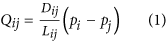where pi is a pressure at a node Ni, Dij is a conductivity of a tube Mij and Lij is its length.

By considering that the inflow and outflow must be balanced, we have: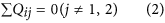For the source node N1 and the sink node N2 the following two equations hold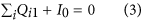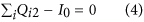where I0 is the flux flowing from the source node and I0 is a constant value here.

In order to describe such an adaptation of tubular thickness we assume that the conductivity Dij changes over time according to the flux Qij. An evolution of Dij(t) can be described by the following equation: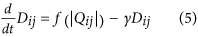where γ is a decay rate of the tube. The equation implies that a conductivity becomes nil if there no flux along the edge. The conductivity increases with the flux. The f is monotonically increasing continuous function satisfying f(0) = 0.

Then the network Poisson equation for the pressure can be obtained from the Eq. (1)-(4), as follows:By setting p2 = 0 as a basic pressure level, all pi can be determined by solving Eq. (6) and Qij can also be obtained.

In this paper,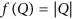is used because, Physarum can always converge to the shortest path regardless of whether the distribution of conductivities in the initial state is random or biased49. With the flux calculated, the conductivity can be derived, where Eq. (7) is used instead of Eq. (5), adopting the functional form.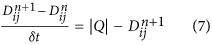Here,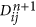represents the conductivity on link (i,j) in the n + 1 iteration. The first part |Q| in the above equation means the acquired energy while the second partdenotes the energy consumed by Physarum. For details, please refer to Ref. 49.

### The Gravity Model

Gravity models are trip distribution models, which have been widely used in transportation systems for estimating the traffic flow between the origins and the destinations57,58,59,60,61,62. The gravity model adapts the concept of the law of universal gravitation: it takes into consideration the population of two different places, corresponding to mass in gravity and the distance between them. The gravity model can be expressed in the following form: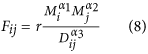where Fij represents the traffic flow starting from node i to node j; Mi and Mj denote the economic sizes of these two places, respectively; Dij is an economic cost associated with these two positions, such as the distance between them, G is an index and it has a constant value. Here, the traffic flow for an individual city is meant to the sum of outward traffic flow.

In the past decades, many researchers have shown that the traffic flow is highly dependent on Gross Domestic Product (GDP) of the associated areas. For example, Cline et al.63 demonstrated that there was a positive relationship between GDP and freight traffic. Zhang and Guo64 found that the air traffic flow of Beijing International Airport and its corresponding GDP was positively correlated with the correlation coefficient up to 0.968. From this point of view, GDP can be used to predict the actual traffic flow. Except that, GDP is a comprehensive indicator. In this indicator, it has accounted for many factors, such as population, industries, income, etc, which in turn reflects development level of a city. As a result, it is more comprehensive in comparison with population. Hence, we will use GDP to represent the economic sizes of the cities.

On the other hand, with the rapid development of transportation networks, including air traffic networks, railways networks and highway networks, the world has become smaller than before. In this case, the factor distance is not so important as before. For example, Marimoutou et al.65 explicitly stated that The larger the partner’s GDP, the less will be the distance effect on trade . Kwon and Jung59 revealed that the total bus flows between cities depends on only its population size. As a result, we assume that the traffic flow depends on the square root of the product of the GDP of city A and the GDP of city B, but has no relation with the distance between them. As a result, α12 are set the same value 0.5. At the same time, as traffic flow has no relation with the distance between these cities, as a result, α3 is 0.

In order to confirm our assumption, we have compared our model with real traffic data and the classical gravity model for the traffic flow of China in 2011. In classical gravity model, they predict the traffic flow as the square root of the product of the population of city A and city B over the square of the distance between them. Based on the data shown in Table 2, by normalizing the traffic flow, we display the traffic flow prediction results between these models in Fig. 10. As can be noted that, the proportions traffic flow trends uncovered in our model and observed in the real traffic data are similar. We can change the prediction results proportionally by adjusting the value of r existing in Eq. (8). However, for the classical gravity model, there are obvious differences between the prediction results and the real traffic data. For example, in the classical gravity model, Nanjing has the biggest traffic flow while in real traffic data, Guangzhou has the highest traffic volume. This in turn demonstrates the correctness of our assumption and the efficiency of our method.

### Physarum Model for Network Design Problem

Consider a network G(V,E), where V denotes a set of nodes, E represents a set of arcs, Lij represents the length of edge (i,j). Assume F is a set of traffic flow in the network G and Fij denotes the traffic flow from the origin i to the destination j. Ft represents a number of O-D pairs (Here, the O-D pairs denotes the table of origin-destination demand) in F. Here, Eq. (6) is expressed in the following form: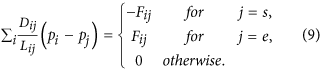Next, nodes i and j are starting and ending node in the Physarum model, respectively. The Physarum algorithm runs only for one iteration when i = j.

Each link in the network is filled with some flux and their conductivity changes correspondingly. As assume the links obtain some energy from the flux whilst some energy will be also consumed. We employ Physarum to simulate the traffic flow onthe link Fik (i,k) E, the procedure is similar with that of traffic flow Fij. Energy in the network is limited. Therefore, all the links compete with each other for traffic. Unused links gradually fade and disappear.

At this step, we record the conductivity matrix Dkij, which expresses the conductivity matrix when the algorithm of Physarum starting from node i to node j is iterated for kth times. The conductivity matrix of other O-D pairs in the kth iteration can be retained and they are expressed as. To reflect the functioneach O-D pair plays in the network, the following Eq. (10) is constructed.To achieve convergence of the Physarum algorithm, we must keep a scale of the conductivity matrix D ranging from 0 to 1. As a result, the following normalised measure is obtained: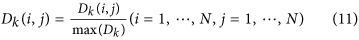where max(Dk) expresses the largest value in the conductivity matrix Dk.

In what follows, Dk will be input as the initialised value of the conductivity matrix for the (k + 1)th iteration. The algorithm runs until a termination criterion is met. There could be several possible termination criteria, including a maximum number of iterations achieved or stationary flux through each tube recorded. In present paper, we adopt the following termination criterion: the algorithm stops when values of conductivity matrix elements stabilize. A general flow of this method is shown in Algorithm 1.

How to cite this article: Zhang, X. et al. A Biologically Inspired Network Design Model. Sci. Rep. 5, 10794; doi: 10.1038/srep10794 (2015).

## References

1. Chen, L., & Yang, H. Managing congestion and emissions in road networks with tolls and rebates. Transp. Res. Part B: Methodological 46, 933–948 (2012).

2. Baublys, A., & Išoraitė, M. Improvement of external transport cost evaluation in the context of Lithuanias integration into the European Union. Transport Reviews 25, 245–259 (2005).

3. Yang, H., & Wang, X. Managing network mobility with tradable credits. Transp. Res. Part B: Methodological 45, 580–594 (2011).

4. Zhang, X., Yang, H., & Huang, H. J. Improving travel efficiency by parking permits distribution and trading. Transp. Res. Part B: Methodological 45, 1018–1034 (2011).

5. Nie, Y. M. Transaction costs and tradable mobility credits. Transp. Res. Part B: Methodological 46, 189–203 (2012).

6. Xiao, F., Qian, Z. S., & Zhang, H. M. Managing bottleneck congestion with tradable credits. Transp. Res. Part B: Methodological 56, 1–14 (2013).

7. Wang, X., & Yang H. Bisection-based trial-and-error implementation of marginal cost pricing and tradable credit scheme. Transp. Res. Part B: Methodological 46, 1085–1096 (2012).

8. Berger, R. T., & Raghavan, S. Long-distance access network design. Manage. Sci. 50, 309–325 (2004).

9. Balakrishnan, A., Magnanti, T. L., & Mirchandani, P. A dual-based algorithm for multi-level network design. Manage. Sci. 40, 567–581 (1994).

10. D’Andreagiovanni, F., Carlo M., & Antonio S. Gub covers and power-indexed formulations for wireless network design. Manage. Sci. 59, 142–156 (2013).

11. Pishvaee, M. S., Farahani, R. Z., & Dullaert, W. A memetic algorithm for bi-objective integrated forward/reverse logistics network design. Comput. Oper. Res. 37, 1100–1112 (2010).

12. Crainic, T. G. Service network design in freight transportation. Eur. J. Oper. Res. 122, 272–288 (2000).

13. Verter, V., & Kara, B. Y. A path-based approach for hazmat transport network design. Manage. Sci. 54, 29–40 (2008).

14. Mauttone, A., & Urquhart, M. E. A route set construction algorithm for the transit network design problem. Comput. Oper. Res. 36, 2440–2449 (2009).

15. Dionne, R., & Florian, M. Exact and approximate algorithms for optimal network design. Networks 9, 37–59 (1979).

16. Gabrel, V., Knippel, A., & Minoux, M. Exact solution of multicommodity network optimization problems with general step cost functions. Oper. Res. Lett. 25, 15–23 (1999).

17. Davis, G. A. Exact local solution of the continuous network design problem via stochastic user equilibrium assignment. Transp. Res. Part B: Methodological 28, 61–75 (1994).

18. Dressler, F., & Akan, O. B. Bio-inspired networking: from theory to practice. IEEE Commun. Mag. 48, 176–183 (2010).

19. Juang, C. F. A hybrid of genetic algorithm and particle swarm optimization for recurrent network design. IEEE Trans. on Cybern. 34, 997–1006 (2004).

20. Fan, W., & Machemehl, R. B. Optimal transit route network design problem with variable transit demand: genetic algorithm approach. J. Transp. Eng. 132, 40–51 (2006).

21. Lo, C. C., & Chang, W. H. A multiobjective hybrid genetic algorithm for the capacitated multipoint network design problem. IEEE Trans. on Cybern. 30, 461–470 (2000).

22. Barbarossa, S., & Scutari, G. Bio-inspired sensor network design. Signal Processing Mag. 24, 26–35 (2007).

23. Das, T. K., Venayagamoorthy, G. K., & Aliyu, U. O. Bio-inspired algorithms for the design of multiple optimal power system stabilizers: SPPSO and BFA. IEEE Trans. Ind. Appl. 44, 1445–1457 (2008).

24. Wolfe, W. J., & Sorensen, S. E. Three scheduling algorithms applied to the earth observing systems domain. Manage. Sci. 46, 148–166 (2000).

25. Singh, P., Sreenivasan, S., Szymanski, B. K., & Korniss, G. Threshold-limited spreading in social networks with multiple initiators. Sci. Rep. 3, 10.1038/srep02330, (2013).

26. Hébert-Dufresne, L., Allard, A., Young, J. G., & Dubé, L. J. Global efficiency of local immunization on complex. Sci. Rep. 3, 10.1038/srep02171, (2013).

27. Juang, C. F., & Chang, P. H. Designing fuzzy-rule-based systems using continuous ant-colony optimization. IEEE Trans. Fuzzy Syst. 18, 138–149 (2010).

28. Poorzahedy, H., & Rouhani, O. M. Hybrid meta-heuristic algorithms for solving network design problem. Eur. J. Oper. Res. 182, 578–596 (2007).

29. Tom, V. M., & Mohan, S. Transit route network design using frequency coded genetic algorithm. J. Transp. Eng. 129, 186–195 (2003).

30. Zhang, X. et al. IFSJSP: a novel methodology for the job-shop scheduling problem based on intuitionistic fuzzy sets. Int. J. Prod. Res. 51, 5100–5119 (2013).

31. Wang, C., Yu, S., Chen, W., & Sun, C. Highly efficient light-trapping structure design inspired by natural evolution. Sci. Rep. 3, 10.1038/srep01025, (2013).

32. Su, Z. et al. Robustness of interrelated traffic networks to cascading failures. Sci. Rep. 4, 10.1038/srep05413, (2014).

33. Latora, V., & Marchiori, M. Efficient behavior of small-world networks. Phys. Rev. Lett. 87, 198701 (2001).

34. Bicchi, A., & Pallottino, L. On optimal cooperative conflict resolution for air traffic management systems. IEEE Trans. Intell. Transp. Syst. 1, 221–231 (2000).

35. Dickinson, M. H. et al. How animals move: an integrative view. Science 288, 100–106 (2000).

36. Nakagaki, T., Yamada, H., & Ágota Tóth, A. Intelligence: Maze-solving by an amoeboid organism. Nature 407, 470–470 (2000).

37. Solnon, C. Ants can solve constraint satisfaction problems. IEEE Trans. Evol. Comput. 6, 347–357 (2002).

38. Detrain, C., Natan, C., Deneubourg, J. L. The influence of the physical environment on the self-organised foraging patterns of ants. Naturwissenschaften 88, 171–174 (2001).

39. Krasnogor, N., Giuseppe, N., Mario, P., & David, P. Nature inspired cooperative strategies for optimization (nicso 2007), Springer, (2008)

40. Stephenson, S. L., Stempen, H., & Hall, I. Myxomycetes: a handbook of slime molds (Timber Press, Oregon, 1994).

41. Nakagaki, T., Yamada, H., & Ueda, T. Interaction between cell shape and contraction pattern in the physarum plasmodium. Biophys. Chem. 84, 195–204 (2000).

42. Nakagaki, T., Yamada, H., & Toth, A. Path finding by tube morphogenesis in an amoeboid organism. Biophys. Chem. 92, 47–52 (2001).

43. Adamatzky, A. Physarum machines: computers from slime mould (World Scientific, Singapore, 2010).

44. Tero, A. et al. Rules for biologically inspired adaptive network design. Science 327, 439–442 (2010).

45. Adamatzky, A. Physarum machine: implementation of a kolmogorov-uspensky machine on a biological substrate. Parallel Processing Lett. 17, 455–467 (2007).

46. Shirakawa, T., Adamatzky, A., Gunji, Y. P., & Miyake, Y. On simultaneous construction of voronoi diagram and delaunay triangulation by physarum polycephalum. Int. J. Bifurcation Chaos 19, 3109–3117 (2009).

47. Tsuda, S., Aono, M., & Gunji, Y. P. Robust and emergent physarum logical-computing. Biosystems 73, 45–55 (2004).

48. Schumann, A., & Adamatzky, A. Physarum spatial logic. New Mathematics and Natural Computation 7, 483–498 (2011).

49. Tero, A., Kobayashi, R., & Nakagaki, T. A mathematical model for adaptive transport network in path finding by true slime mold. J. Theor. Biol. 244, 553–564 (2007).

50. Bonifaci, V., Mehlhorn, K., & Varma, G. Physarum can compute shortest paths. J. Theor. Biol. 309, 121–133 (2012).

51. Erlander, S., & Stewart, N. F. The gravity model in transportation analysis: theory and extensions, Vol. 3 (VSP, Netherlands, 1990).

52. Adamatzky, A., Martinez, G. J., Chapa-Vergara, S. V., Asomoza-Palacio, R., & Stephens, C. R. Approximating mexican highways with slime mould. Natural Computing 10, 1195–1214 (2011).

53. Tsompanas, M. A., Sirakoulis, G., & Adamatzky, A. Evolving transport networks with cellular automata models inspired by slime mould. IEEE Trans. on Cybern. 10.1109/TCYB.2014.2361731, (2014).

54. Tsompanas, M. A., Sirakoulis, G., & Adamatzky, A. Physarum in silicon: the Greek motorways study. Nat. Comput. 10.1007/s11047-014-9459-0, (2014).

55. China Population. National Bureau of Statistics of China. http://data.stats.gov.cn/datamap/index?m=fsnd, (2011) (Date of access: 20/08/2014).

56. Johnson, D. S., Lenstra, J. K., & Kan, A. H. G. The complexity of the network design problem. Networks 8, 279–285 (1978).

57. Evans, S. P. A relationship between the gravity model for trip distribution and the transportation problem in linear programming. Transp. Res. 7, 39–61 (1973).

58. Carrere, C. Revisiting the effects of regional trade agreements on trade flows with proper specification of the gravity model. Eur. Econ. Rev. 50, 223–247 (2006).

59. Kwon, O., & Jung, W. S. Intercity express bus flow in korea and its network analysis. Physica A 391, 4261–4265 (2012).

60. Simini, F., González, M. C., Maritan, A., & Barabási, A. L. A universal model for mobility and migration patterns. Nature 484, 96–100 (2012).

61. Simini, F., Maritan, A. & Néda, Z. Human mobility in a continuum approach. Plos one 8, e60069 (2013).

62. Ren, Y., Ercsey-Ravasz, M., Wang, P., González, M. C., & Toroczkai, Z. Predicting commuter flows in spatial networks using a radiation model based on temporal ranges. Nat. Commun. 5 10.1038/ncomms6347, (2014).

63. Cline, R. C., Ruhl, T. A., Gosling, G. D., & Gillen, D. W. Air transportation demand forecasts in emerging market economies: a case study of the Kyrgyz Republic in the former Soviet Union. J. Air Transport Manage. 4, 11–23 (1998).

64. Zhang, Z., & Guo, S. Gray interval prediction of air traffic flow of capital airport. J. Civil Aviat. Univ. of China 25, 1–4 (2007).

65. Marimoutou, V., Peguin, D., & Peguin-Feissolle, A. The” distance-varying” gravity model in international economics: is the distance an obstacle to trade? Economics Bulletin 29, 1157–1173 (2009).

66. Adamatzky, A., Yang, X. S., & Zhao, Y. X. Slime mould imitates transport networks in China. Int. J. Intell. Comput. and Cybern. 6, 232–251 (2013).

## Acknowledgements

The work is partially supported Chongqing Natural Science Foundation, Grant No. CSCT, 2010BA2003, National Natural Science Foundation of China, Grant Nos. 61174022 and 71271061, National High Technology Research and Development Program of China (863 Program) (No.2013AA013801), Science and Technology Planning Project of Guangdong Province, China (2010B010600034, 2012B091100192), Business Intelligence Key Team of Guangdong University of Foreign Studies (TD1202), Doctor Funding of Southwest University Grant No. SWU110021.

## Author information

Authors

### Contributions

X.Z., A.A., F.T.S.C., S.M. designed and performed research. X.Z. wrote the paper. Y.H., M.A.T., G.S. performed the computation. X.S.Y. and Y.D. analyzed the data. All authors discussed the results and commented on the manuscript.

## Ethics declarations

### Competing interests

The authors declare no competing financial interests.

## Rights and permissions

Reprints and Permissions

• ### An Accelerated Physarum Solver for Network Optimization

• Cai Gao
• , Xiaoge Zhang
• , Zhiying Yue
•  & Daijun Wei

IEEE Transactions on Cybernetics (2020)

• ### A Physarum-Inspired Decision-Making Strategy for Multisource Task Searching of Mobile Robots

• Laihao Jiang
• , Hongwei Mo
•  & Lifang Xu

Complexity (2020)

• ### A parallel bio-inspried shortest path algorithm

• Hilal Arslan
•  & Murat Manguoglu

Computing (2019)

• ### Lessons from a virtual slime: marginal mechanisms, minimal cognition and radical enactivism

• Lachlan Douglas Walmsley

• ### Optimizing biologically inspired transport networks by control

• Junjie Jiang
• , Xingang Wang
•  & Ying-Cheng Lai

Physical Review E (2019)